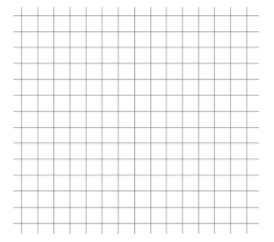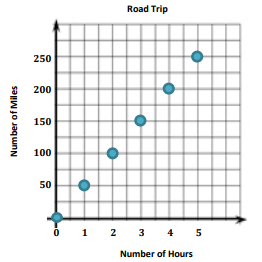# Equations and Inequalities

## Objective

Represent the relationship between two quantities in graphs, equations, and tables. (Part 1)

## Common Core Standards

### Core Standards

?

• 6.EE.C.9 — Use variables to represent two quantities in a real-world problem that change in relationship to one another; write an equation to express one quantity, thought of as the dependent variable, in terms of the other quantity, thought of as the independent variable. Analyze the relationship between the dependent and independent variables using graphs and tables, and relate these to the equation. For example, in a problem involving motion at constant speed, list and graph ordered pairs of distances and times, and write the equation d = 65t to represent the relationship between distance and time.

• 6.RP.A.3.A — Make tables of equivalent ratios relating quantities with whole number measurements, find missing values in the tables, and plot the pairs of values on the coordinate plane. Use tables to compare ratios.

?

• 5.OA.B.3

• 6.NS.C.6.C

## Criteria for Success

?

1. Identify the independent and dependent variables in a relationship between two quantities.
2. Create a table of values for a relationship.
3. Plot values from a table on a coordinate plane.
4. Write equations to represent the relationship between two associated quantities.
5. Interpret points in a coordinate plane in context of the situation.

## Tips for Teachers

?

Lessons 13 and 14, similar to Lesson 12, bring together several concepts from standards such as 6.RP.3, 6.EE.7, and 6.NS.8 to represent relationships between quantities in different ways. In this lesson, students look at proportional relationships in the form ${px=q}$.

#### Fishtank Plus

• Problem Set
• Student Handout Editor
• Vocabulary Package

## Anchor Problems

?

### Problem 1

Sarah is purchasing pencils to share. Each package has 12 pencils. The equation ${n=12p}$, where $n$ is the total number of pencils and $p$ is the number of packages, can be used to determine the total number of pencils Sarah purchased.

1. Determine which variable is dependent and which variable is independent.
2. Make a table showing the number of pencils for 3–7 packages.
3. Plot the points in the coordinate plane.
4. If Sarah has 168 pencils, how many packages did she purchase?
 # of packages ($p$) Total # of pencils ($n$)#### References

EngageNY Mathematics Grade 6 Mathematics > Module 4 > Topic H > Lesson 31Exercise 1

Grade 6 Mathematics > Module 4 > Topic H > Lesson 31 of the New York State Common Core Mathematics Curriculum from EngageNY and Great Minds. © 2015 Great Minds. Licensed by EngageNY of the New York State Education Department under the CC BY-NC-SA 3.0 US license. Accessed Dec. 2, 2016, 5:15 p.m..

Modified by The Match Foundation, Inc.

### Problem 2

The graph below shows the relationship between the number of miles, $m$, and number of hours, $h$, spent traveling on Veronica’s road trip.1. Which variable is the independent variable? The dependent variable?
2. What does the point (4, 200) mean in context of the situation?
3. Describe the relationship between the two quantities.
4. Write an equation to represent the relationship.

#### References

EngageNY Mathematics Grade 6 Mathematics > Module 4 > Topic H > Lesson 32Example 2

Grade 6 Mathematics > Module 4 > Topic H > Lesson 32 of the New York State Common Core Mathematics Curriculum from EngageNY and Great Minds. © 2015 Great Minds. Licensed by EngageNY of the New York State Education Department under the CC BY-NC-SA 3.0 US license. Accessed Dec. 2, 2016, 5:15 p.m..

Modified by The Match Foundation, Inc.

### Problem 3

At a store closing, everything in the store is 50% its original price.

1. Write an equation to represent the discounted price, $d$, of any item in the store with an original price $p$.
2. Create a table of values with at least three or four different original prices.
3. Plot the values in the coordinate plane to show the relationship between the original price and the discounted price of items in the store.
4. Explain how you could use your graph to find the discounted price of an item that originally cost 15.  Original Price ($p$) Discounted Price ($d$)#### Guiding Questions Create a free account or sign in to access the Guiding Questions for this Anchor Problem. ## Problem Set ?With Fishtank Plus, you can download a complete problem set and answer key for this lesson. Download Sample The following resources include problems and activities aligned to the objective of the lesson that can be used to create your own problem set. ## Target Task ? Arian wants to save 20% of his paychecks in a savings account. 1. Write an equation to represent the amount Arian should save, $s$, from a paycheck in the amount of $p$ dollars. 2. Create a table of values with at least three or four different paycheck amounts. 3. Plot the values in the coordinate plane to show the relationship between the amount Arian saves and the amount Arian earns. 4. Explain how you could use your graph to find how much of a60 paycheck Arian should put into his savings account.
 Paycheck Amount ($p$) Savings Amount ($s$)?